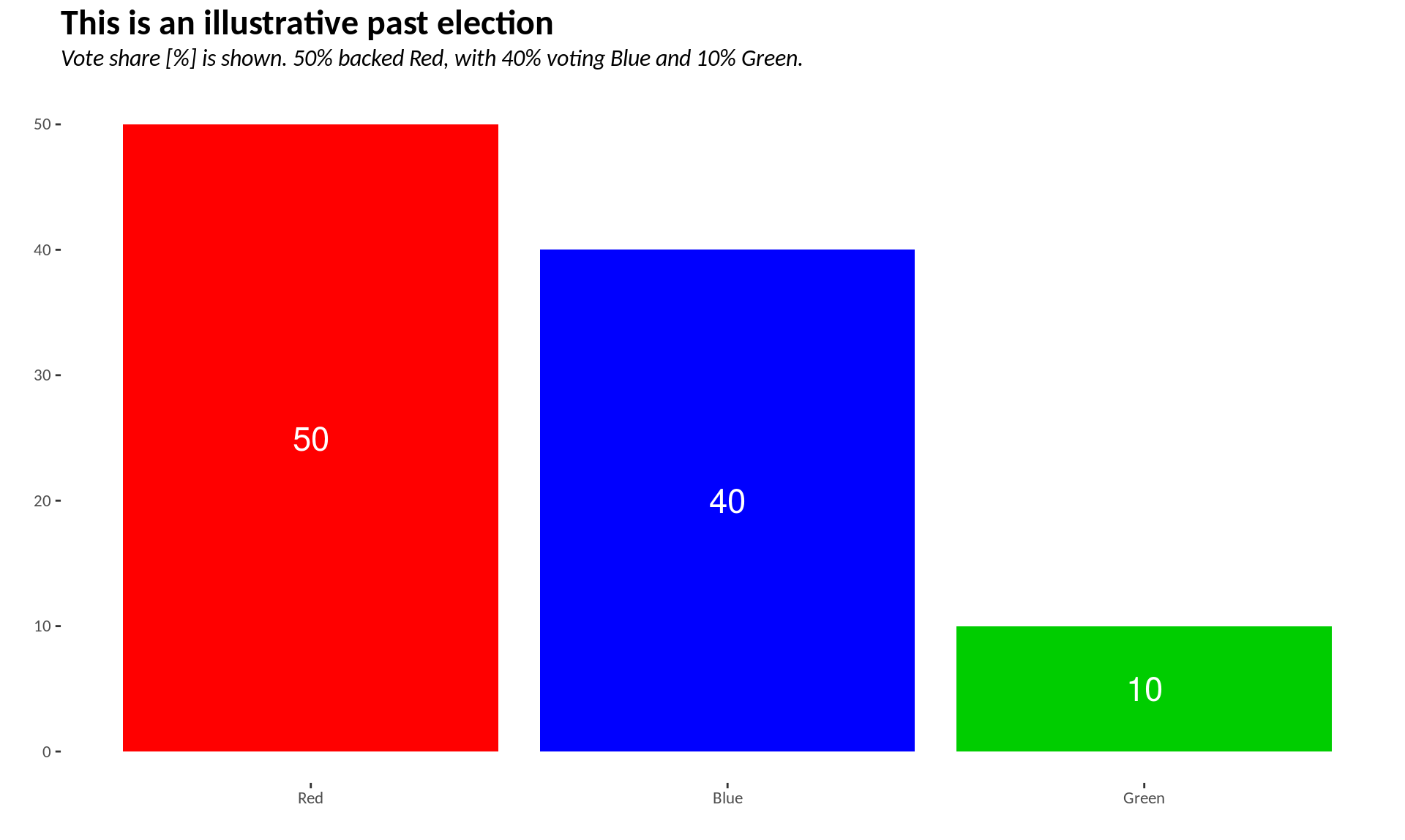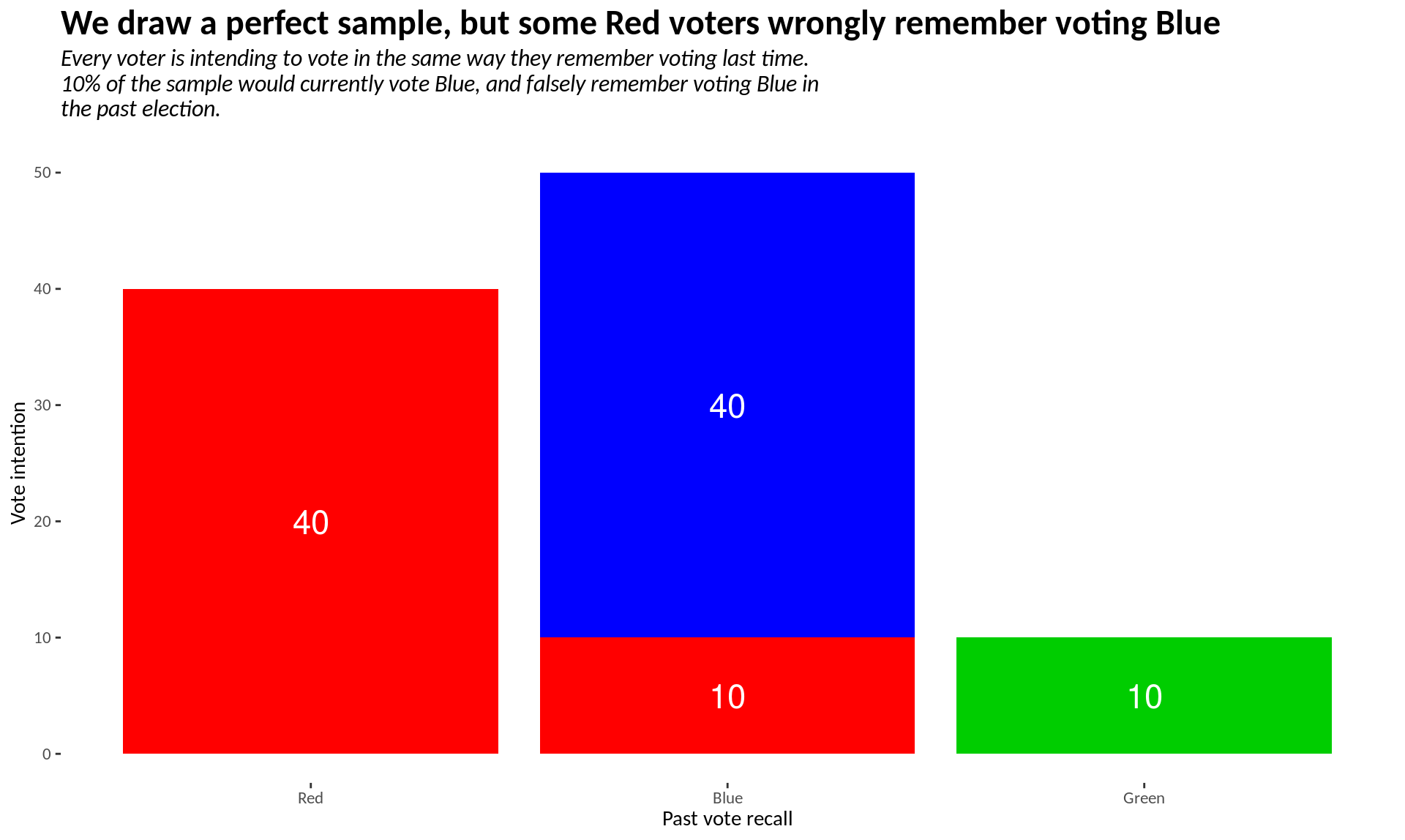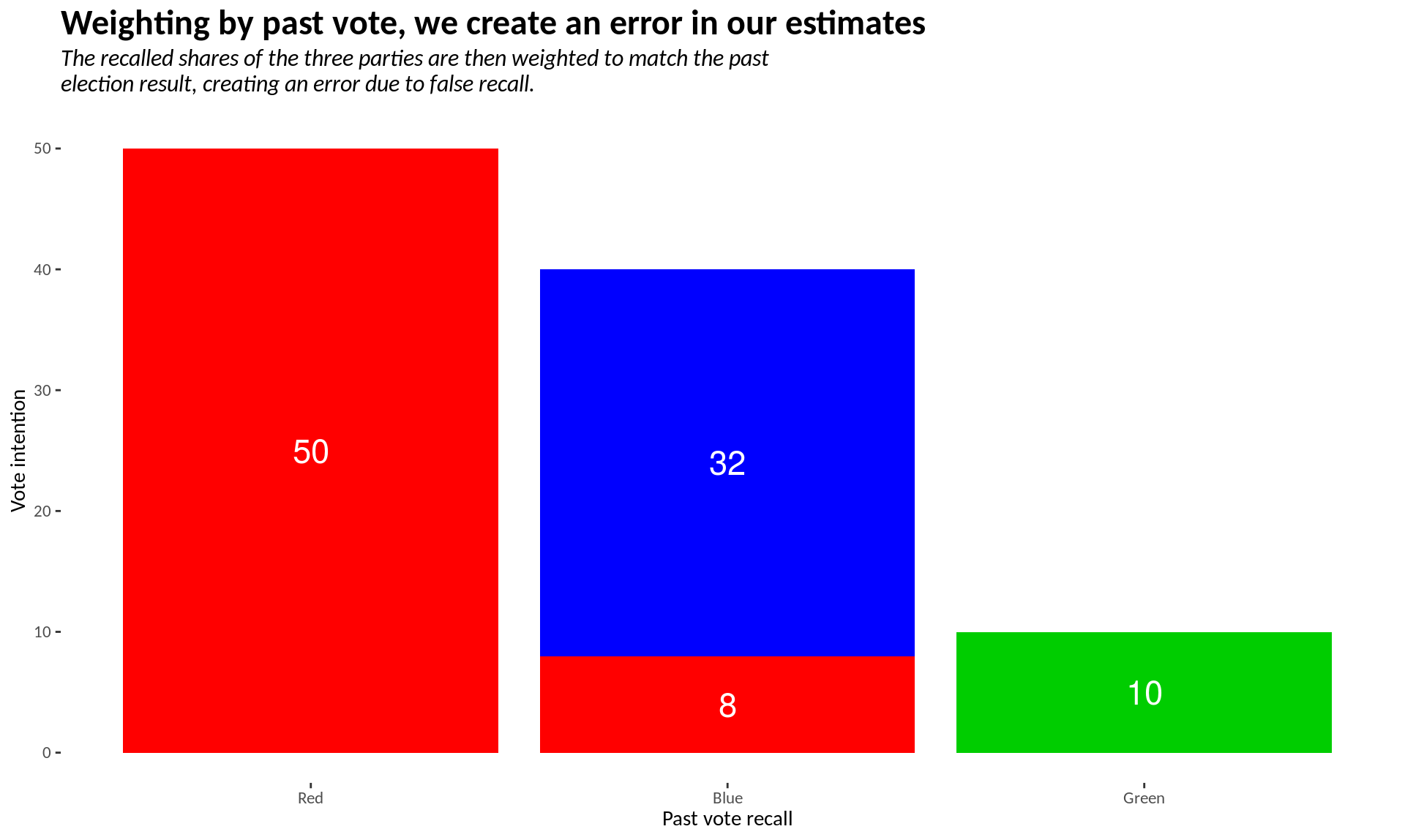## R Markdown

The purpose of this code to create three graphs, illustrating the issue of false recall and past vote weighting in surveys.

## Packages and Themes

First, we install the tidyverse package.

library(tidyverse)

Next, we create our custom theme.

theme_clean <- theme_bw(base_family="Calibri") +
theme(legend.position = "top",
legend.title = element_text(size = 12),
legend.text = element_text(size = 12),
plot.title = element_text(size = 18, face = "bold"),
plot.subtitle = element_text(size = 12, face = "italic", margin = margin(b=12)),
plot.caption = element_text(size = 10),
panel.grid.major = element_blank(),
panel.grid.minor = element_blank(),
panel.border = element_blank())
theme_set(theme_clean)

## Create data tables

The three tables are manually inputted as tribbles.

pastresult_df <- tribble(~Party, ~Share,
"Red", 50,
"Blue", 40,
"Green", 10)
currentVI_df <- tribble(~Past, ~Current, ~Share,
"Red", "Red", 40,
"Blue", "Blue", 40,
"Red", "Blue", 10,
"Green", "Green", 10)
currentVIweighted_df <- tribble(~Past, ~Current, ~Share,
"Red", "Red", 50,
"Blue", "Blue", 32,
"Red", "Blue", 8,
"Green", "Green", 10)

Additionally, I set factors so the parties appear in the ‘right’ order.

pastresult_df$Party <- factor(pastresult_df$Party,
levels = c("Red", "Blue", "Green"))
currentVI_df$Current <- factor(currentVI_df$Current,
levels = c("Red", "Blue", "Green"))
currentVIweighted_df$Current <- factor(currentVIweighted_df$Current,
levels = c("Red", "Blue", "Green"))

## Create the graphs

Finally, we create our three graphs.

pastresult_gg <- ggplot(data = pastresult_df,
aes(x = Party, y = Share, fill = Party)) +
geom_bar(stat = "identity") +
labs(x = "", y = "",
title = "This is an illustrative past election",
subtitle = str_wrap("Vote share [%] is shown. 50% backed Red, with 40% voting Blue and 10% Green.")) +
theme(legend.position = "none") +
scale_fill_manual(values = c("red", "blue", "green3")) +
geom_text(aes(label = Share),
position = position_stack(vjust = 0.5),
colour = "white", size = 6)

This is the first graph:

pastresult_ggcurrentVI_gg <- ggplot(data = currentVI_df,
aes(x = Current, y = Share, fill = Past)) +
geom_bar(position = "stack", stat = "identity") +
labs(x = "Past vote recall", y = "Vote intention",
title = "We draw a perfect sample, but some Red voters wrongly remember voting Blue",
subtitle = str_wrap("Every voter is intending to vote in the same way they remember voting last time. 10% of the sample would currently vote Blue, and falsely remember voting Blue in the past election.")) +
theme(legend.position = "none") +
scale_fill_manual(values = c("blue", "green3", "red")) +
geom_text(aes(label = Share),
position = position_stack(vjust = 0.5),
colour = "white", size = 6)

This is the second graph:

currentVI_ggcurrentVIweighted_gg <- ggplot(data = currentVIweighted_df,
aes(x = Current, y = Share, fill = Past)) +
geom_bar(position = "stack", stat = "identity") +
labs(x = "Past vote recall", y = "Vote intention",
title = "Weighting by past vote, we create an error in our estimates",
subtitle = str_wrap("The recalled shares of the three parties are then weighted to match the past election result, creating an error due to false recall.")) +
theme(legend.position = "none") +
scale_fill_manual(values = c("blue", "green3", "red")) +
geom_text(aes(label = Share),
position = position_stack(vjust = 0.5),
colour = "white", size = 6)

This is the third graph:

currentVIweighted_gg